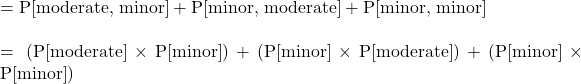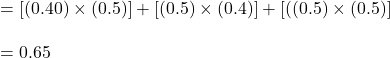## Workplace accidents are categorized in three groups: minor, moderate, and severe. The probability that a given accident is minor is 0.5, tha

Question

Workplace accidents are categorized in three groups: minor, moderate, and severe. The probability that a given accident is minor is 0.5, that it is moderate is 0.4, and that it is severe is 0.1. Two accidents occur independently in one month. Calculate the probability that neither accident is severe and at most one is moderate.

in progress 0
2 weeks 2021-09-05T15:49:13+00:00 1 Answers 0 views 0

1. Answer: The probability when neither of the accidents is severe and at most one is moderate is 0.65

Step-by-step explanation:

Given values:

Probability when the accident is minor = 0.5

Probability when the accident is moderate = 0.4

Probability when the accident is severe = 0.1

As two accidents are occurring independently and we need to calculate the probability of an event that neither accident is severe and at most one is moderate.

So, the equation for the probability becomes:Putting values in above equation, we get:Hence, the probability when neither of the accidents is severe and at most one is moderate is 0.65